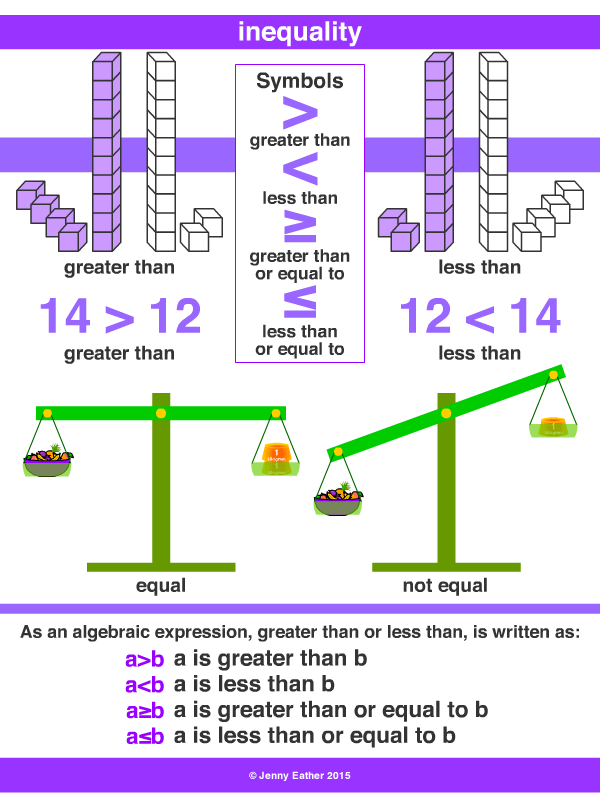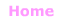Aa Bb Cc Dd Ee Ff Gg Hh Ii Jj Kk Ll Mm Nn Oo Pp Qq Rr Ss Tt Uu Vv Ww Xx Yy Zz

Ii

inequality

• inequality occurs when things are not equal.
• there are four types of inequality:
a>b … a is greater than b
a<b … a is less than b
a≥b … a is greater than or equal to b
a≤ba … a is less than or equal to b

EXAMPLES:Properties of inequality

Exactly one of the following is true
a < b, a = b, a > b.

If a > b and b > c then a > c.

If a > b, then b < a.

If a > b, then –a < –b.

If a > b, then a ± c > b ± c.

If a > b and c > 0, then a × c > b × c.

If a > b and c < 0, then a × c < b × c.

If a > b and c > 0, then a ÷ c > b ÷ c.

If a > b and c < 0, then a ÷ c < b ÷ c.© Jenny Eather 2014. All rights reserved.